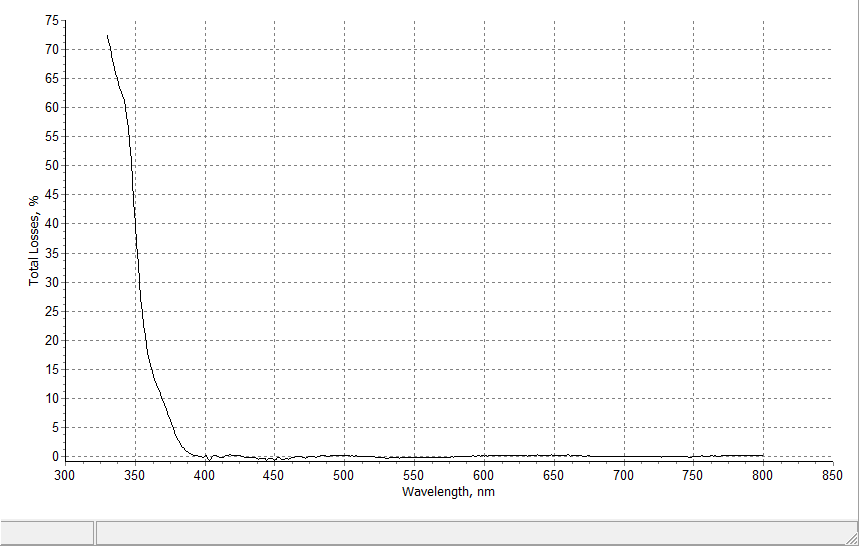### Total Losses

 Total losses option allows estimating the total losses values in thin film samples. These values are calculated using simple formula $A(\lambda)-100\%-T(\lambda)-R(\lambda)$ In order to display these values current measurement data should include both reflectance and transmittance values for the common wavelength region. Sometimes oscillations in total losses can be observed. The reasons of such oscillations are carefully studied in our paper: T. Amotchkina, M. Trubetskov, A. Tikhonravov, V. Janicki, J. Sancho-Parramon, O. Razskazovskaya, and V. Pervak, "Oscillations in spectral behavior of total losses (1 - R - T) in thin dielectric films," Opt. Express 20, 16129-16144 (2012).